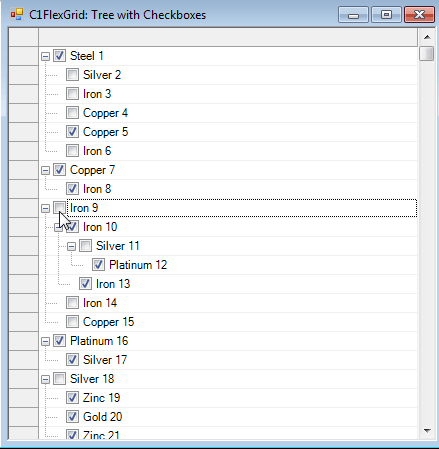﻿ Check/Uncheck All Child Nodes by Checking/Unchecking Root Node in TreeView FlexGrid# Check/Uncheck All Child Nodes by Checking/Unchecking Root Node in TreeView FlexGrid

The C1FlexGrid can group data hierarchically and display it with a collapsible tree (similar to the one in the Microsoft TreeView control). The GridTree object is used to specify the position and appearance of the outline tree. We can also show CheckBoxes for each treenode. Extending this functionality we can add a feature which enables us to check/uncheck child nodes when the root/parent node is checked/unchecked. Through this blog we will learn how to implement this feature in a C1FlexGrid control.### Binding C1Flexgrid and Creating a Custom Tree

We will create a random collection of treenodes for the C1Flexgrid. In effect, every time the sample is run a different grid is shown. We can also implement the same functionality on a bound grid as well. Also, we will set the checkboxes for each node and set the checked state of each node. Code

``````private void Form1_Load(object sender, EventArgs e)
{
_flex.Cols.Width = 30;
_flex.Cols.Count = 2;
_flex.ExtendLastCol = true;
_flex.Tree.Column = 1;

Random r = new Random();
string[] s = "Iron|Steel|Titanium|Gold|Silver|Platinum|Copper|Zinc|Bronze".Split('|');
int level = 0;
_flex.Rows.Count = 1;
for (int i = 1; i  .5) ? CheckEnum.Checked : CheckEnum.Unchecked);

// update level for next node
if (level > 0 && r.NextDouble() > .75) level--;
else if (level == 0 || r.NextDouble() > .8) level  ;
}
}
``````

Run the application and you will see a Custom Tree C1FlexGrid bound to a random collection.

### Set CheckState for Child Nodes through Root Node

This step is even easier then the first one. We will handle the CellChecked event of C1Flexgrid. The e.Row property would return the node being checked. We will get the count of children for this node using _flex.Rows[e.Row].Node.Children. If the number of children is greater than zero, we will create an object of CellRange which will store the CellRange of this tree node. The firstrow of this range would be the CellRange.r1 and the lastrow would be CellRange.r2 property. Now we will traverse through this cellrange from the first row to the last row and based on the checked state of e.Row, we will check the nodes in this cell range. Code

``````private void \_flex\_CellChecked(object sender, RowColEventArgs e)
{
int children = _flex.Rows[e.Row].Node.Children;
int firstRow, lastRow;

if (children > 0)
{
CellRange cr = _flex.Rows[e.Row].Node.GetCellRange();
firstRow = cr.r1;
lastRow = cr.r2;

CheckEnum ck = _flex.GetCellCheck(e.Row, e.Col);

for (int i = firstRow; i <= lastRow; i  )
_flex.SetCellCheck(i, e.Col, ck);
}
}

``````